#### A breakdown of the mathematical intuition behind GCNs.

This is the third part of a series on graphs and graph theory in machine learning.

By now, if you’ve been following this series, you may have learned a bit about graph theory, why we care about graph structured data in data science, and what the heck a “Graph Convolutional Network” is. Now, I’d like to briefly introduce you to what makes these things work.

For my friends in the field who may know a bit about this subject, I’ll be talking about the intuition behind Kipf & Welling’s 2017 paper on graph convolutions for semi-supervised learning (Thomas Kipf wrote a more digestible explanation of this concept here), although other approaches have been developed since improving on this methodology. My goal is to introduce the mathematical concepts, rather than provide an extensive overview of the topic.

Now, if you aren’t a mathematician, data scientist, or even a computer scientist, don’t run away just yet. The goal is to take the academic papers and break down the concepts so that anyone can understand. Feel free to leave a reply at the end letting me know if I’ve achieved this goal or not!

#### Let’s Get Going! — GCNs, What’s the Goal?

In Thomas N. Kipf’s blog post, he says the goal of a Graph Convolutional Network is to:

“…learn a function of signals/features on a graph G =(v, e)”

But what does that even mean? Let’s break it down.

There are different methods for performing some prediction or classification on graphs or nodes. But we’re focused on Graph Convolutional Networks, for classification on graphs*. This means, to provide a concrete example, that for something like a protein, which has a structure represented by an entire graph: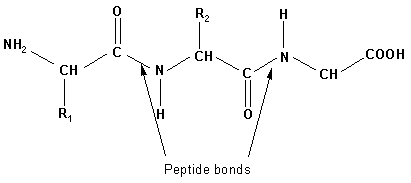Basic structure of a protein, where multiple amino acids are chained via peptide bonds, courtesy of source.

We might want a way to embed this graph’s features such that we could classify what protein we’re looking at.

So, let’s return to our quote above. We want to learn the function of features on a graph. In other words, we want to learn the relationships between features — in the protein example, that might be specific bond representations between certain atoms, or the atomic representations themselves — given our graph’s specific network structure. In other words: how do combinations of nodes and bonds (edges) impact what protein this is that we’re looking at (target label)?

Disclaimer: this, like many things in this post, is a slight oversimplification. The purpose of this is to share the intuition behind GCNs, and make it accessible. For full explanations and mathematical proofs, I encourage you to dive deeper into the papers cited at the bottom.

Why not a normal Convolutional Neural Network? We’ve spoken a bit in previous articles about the difficulties traditional machine learning & deep learning models face when confronted with arbitrarily structured (non-Euclidean) data. What a GCN allows us to do is embed the graph structure into a two-dimensional representation, overcoming this barrier that we would encounter if we attempted to classify this graph using a traditional CNN.

You can think of embedding like taking a three dimensional molecule model, like this: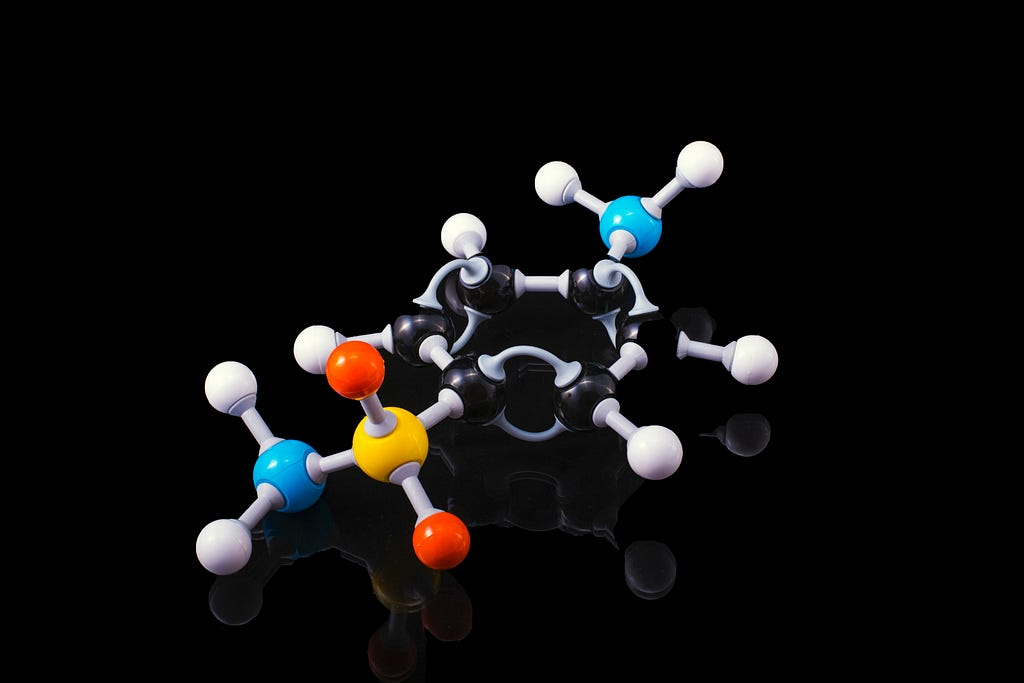Photo courtesy of Terry Vlisidis on Unsplash

And drawing it on a piece of paper, to carry with us and memorize.

#### To Understand How, Let’s Look at Forward Propagation.

I may be projecting my learning style on my readers, but to really fully grasp the formulas that Kipf proposed, I had to go back to basics and review forward propagation. Let’s take a quick second to look at the forward propagation formula: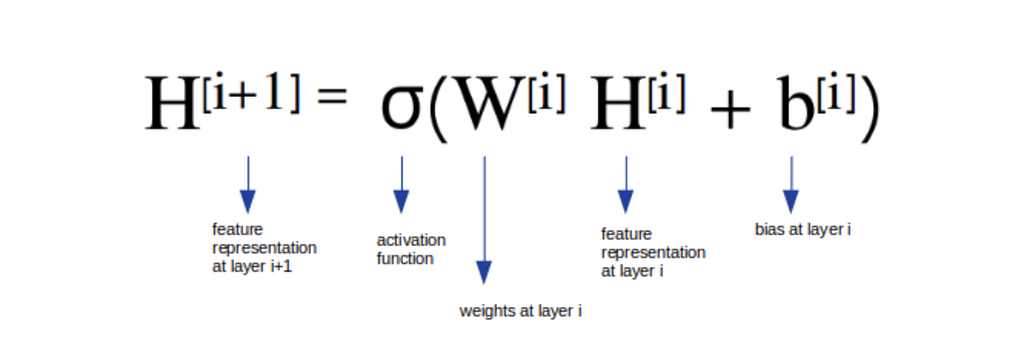Forward propagation equation for regular neural networks. Image courtesy of source

Don’t let this formula scare you. All it’s saying is that the feature representation at the next layer is equal to the result of performing some activation function on the weights at the current layer times the feature representation at the current layer plus the bias at the current layer.

Maybe that still feels dense, and if it does, that’s okay. You can think of this this way: this formula represents is a series of computational steps we take to get to an output, passing the result through each layer in our neural network until i is our final (output) layer. (If you know the basics of programming, you can conceptualize this like a for loop: “for each layer in our neural network’s layers, perform this calculation to return a final value.”)

Our activation function (represented as sigma) might be something like ReLu, and our feature representation can be thought of in terms of our protein example, where each atom in our molecule is represented by, (for the sake of our simple example), its atomic number. We would then want our final output at our last layer to be the representation of what protein we’re looking at — our classification. Don’t worry too much about bias for now if you don’t know what it is, we won’t be discussing it further in this article.

Check-In
It’s possible that if you’re maybe a bit new to all this your brain is hurting right about now, and that’s fine. Let’s take a second to review and digest what we’ve learned before we go further:
1. One goal of a GCN is to take an arbitrarily structured graph and embed it into a two-dimensional representation of a network.
2. Additionally, we want to understand the functions of features on a graph — we want to know how stuff influences other stuff (how features in our graph influence our target).
3. We’ve reviewed forward propagation for regular neural networks, and understand what this equation tells us.

#### Forward Propagation — How Does This Relate to GCNs?

Similar to any neural network, Graph Convolutional Networks need a way to propagate values forward through layers to achieve their goals. However, given that our data is not Euclidean, we’re going to need to make a few slight adjustments to the regular forward propagation equation we discussed above.

First, we need a way to represent our graph computationally. If you remember all the way back to my first article on Graph Theory, or if you remember your mathematics (or, for the computer scientists in the room, your data structures courses), you’ll remember that one way to represent a graph is with an Adjacency Matrix. Let’s take a look at one example: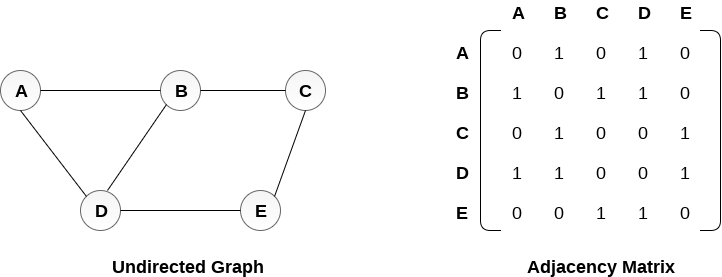Adjacency matrix of an undirected graph, shown on the left.

Our adjacency matrix is effectively a sparse matrix where we have rows and columns representing node labels and a binary representation of whether or not any two nodes are connected to each other. 0 corresponds to “no connection”, where a 1 represents a connection (an edge).

So, we have our first missing piece of the GCN puzzle: our adjacency matrix, which we’ll call A.

Now, we need to make sure we insert A into our propagation equation. Since I said not to worry about bias (and since the article I’m referencing for images also omits this value from its images, this works well), you won’t see it in the following representation.

Let’s take a look at our equation so far:Forward propagation equation for graph convolutional network. Image courtesy of source with my own annotations added.

But we aren’t quite done yet. There are two problems:

1. If we solely look at A, we’ll look at all of a given node’s neighbors, but not that node itself. We’ll deal with this in a moment.
2. What is A*? A, as we initially discussed it, was not normalized. Since we’re going to have to multiply our weights and feature representations by our sparse adjacency matrix A, we can anticipate that the scale of the feature representations and weights will dramatically change with the scale of A. So, A* is normalized A. Let’s talk about what that means:

Kipf & Welling introduced a method to normalize A that takes care of this second problem. First, we need the diagonal node degree matrix, D. If that sentence feels confusing, check out the linked article! One of the ways to normalize A would be to multiply with D, but Kipf & Welling introduce us to another method called symmetric normalization. This equates to: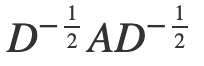Symmetric normalization representation of Adjacency Matrix (A) and Degree Matrix (D), courtesy of source.

You’ll see this in action when we provide our final equation! Now, that we’ve addressed our normalization issue, we’re almost there. We have one more thing to add: self-loops.

We want to learn representations for all of the nodes in our graph, and this means that we need to change something about our graph. Because this is a lengthy article already, I’ll once again quote Thomas Kipf from his blog post to explain:

“… multiplication with A means that, for every node, we sum up all the feature vectors of all neighboring nodes but not the node itself (unless there are self-loops in the graph). We can "fix" this by enforcing self-loops in the graph: we simply add the identity matrix to A.”

Here, we arrive at our next step: enforcing self-loops. This means that we need our nodes to also connect back to themselves, looking something like this: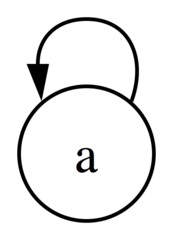Self loop on a single node, courtesy of source.

If you’re not 100% sure what Kipf was talking about in his quote regarding the Identity Matrix, remember that the identity matrix is an n x n matrix with 1’s on the main diagonal (top left to bottom right) and zeroes elsewhere: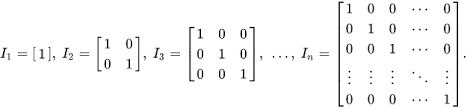Identity matrices n x n, courtesy of source.

Where n is, in our use case, going to be the dimension of our adjacency matrix A.

Now that we’ve normalized A with its degree matrix and enforced self-loops by adding our identity matrix, we arrive at our final equation for forward propagation in graph convolutional networks: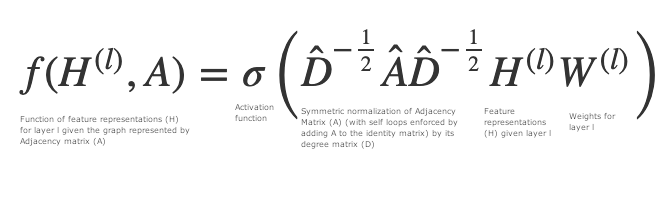Forward propagation equation for graph convolutional networks — equation to derive function of feature representation for layer (l) for adjacency matrix (A), courtesy of source with my own annotations added.

Let’s break down this new equation.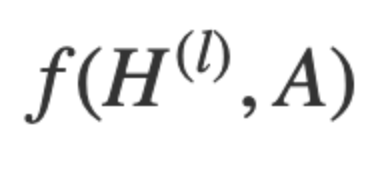First clause of forward propagation equation for GCNs.

This piece says: “The function of our features for layer l given adjacency matrix A”. This is what we’re looking to solve for. Remember one of our original goals, to find the functions of features for a graph.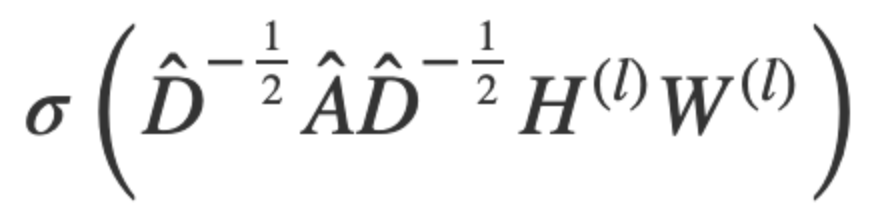Second clause of forward propagation equation for GCNs.

Let’s break this second part down. Remember, this is to the right of the equal sign — this is what we’re using to find the functions of our features.

Remember that sigma (𝝈) represents an activation function (such as ReLu). So what this clause represents is the result of the activation function on the diagonal degree matrix ()* of Â, which is our adjacency matrix (A) added to the identity matrix, which we’ve normalized using “symmetric normalization”. These are then multiplied by our feature representations and our weights for our current layer, l.

Wait — let’s take a step back.

Let’s talk through that last piece piece to our puzzle. Remember how we talked about our protein example, and how our nodes might have a label, like, the name or abbreviation of an atom (i.e: carbon, or C), as well as a feature representation, which might be something like their atomic number?

These representations in this example become our feature representation vectors, and for our equation above, represent H of l.

#### Sum it All Up — In a Few Words

You’ve just learned how to find the functions of features for a given graph, G given its adjacency matrix, A, and its degree matrix, D.

You’ve reviewed forward propagation, and learned how it differs for graph convolutional networks. You’ve learned about some important caveats to consider when dealing with arbitrarily structured data, like the importance of having or creating self-loops and of normalizing our adjacency matrix.

Nice! You’re well prepared to take on the next step — which will be coming soon — and which should be much more fun: actually classifying stuff with GCNs.

If you want to learn even more before next time, check out all of the papers and sources that were so invaluable in the writing of this article:

1. Semi-Supervised Classification with Graph Convolutional Networks, Kipf & Welling (ICLR 2017)
2. Graph Convolutional Networks, Thomas Kipf
3. Understanding Graph Convolutional Networks for Node Classification, Inneke Mayachita

Notes:

1. *GCNs can be used for node-level classification, as well, but we don’t focus on that here, for the sake of a simplified example.
2. *this represents ‘D-hat’, Medium’s mathematical notation support is lacking a bitWhat Makes Graph Convolutional Networks Work? was originally published in Towards Data Science on Medium, where people are continuing the conversation by highlighting and responding to this story.